### Background

• 文档级的关系抽取涉及到多种信息 (non-local/local/syntactic/semantic dependencies), 现有的方法不能充分地利用（exploit）these dependencies.

• Local dependency <=> within sentence 句内依赖

• Dependency parsing 依赖解析
• Non-Local dependencies<=>across sentences 句间依赖

• Co-reference 共指
• Discourse dependencies
• Required for inter-sentence relations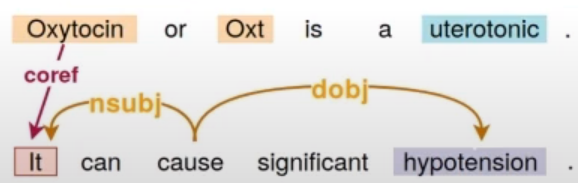• 不同的sentence有不同的 dependency tree.

• 构建==GCNN-based model 捕获 localnon-local dependencies。==
• GCNN : a labelled edge Graph CNN
• 贡献了一个新的数据集
• Effectiveness of local and non-local dependencies on inter-sentence pairs

### Model

IDEA: Utilise local and non-local dependencies in combination.

• Represent a textual snippet as a graph.

• Words = nodes

Edges =local,non-local dependencies

• Incorporate ==GCNN for graph encoding==

• Multi-instance Learning for concept-level relation extraction.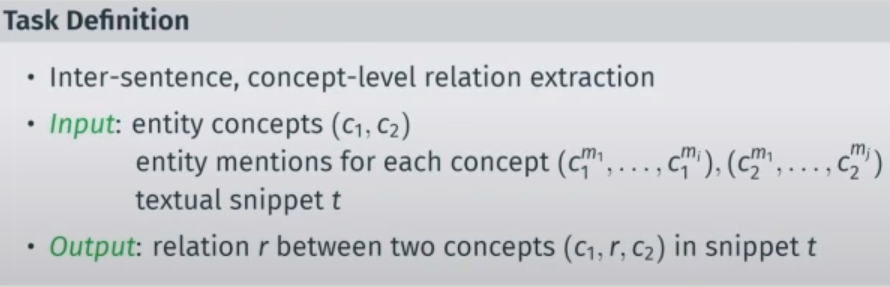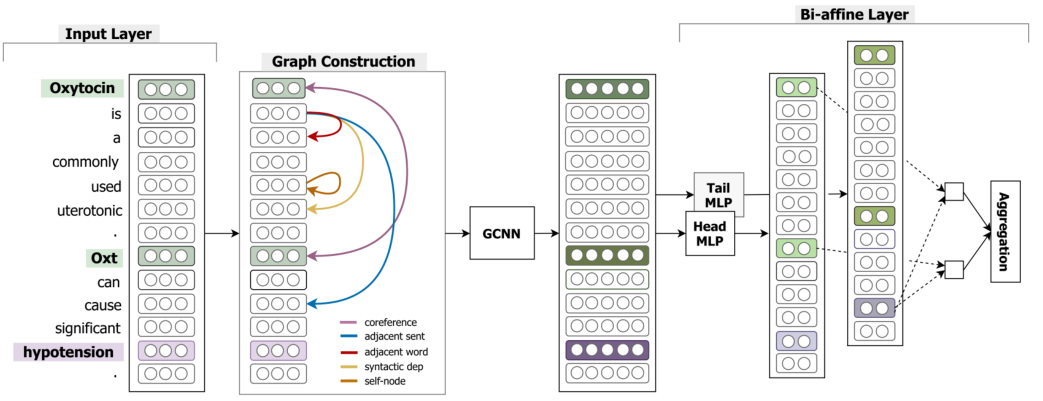Input layer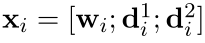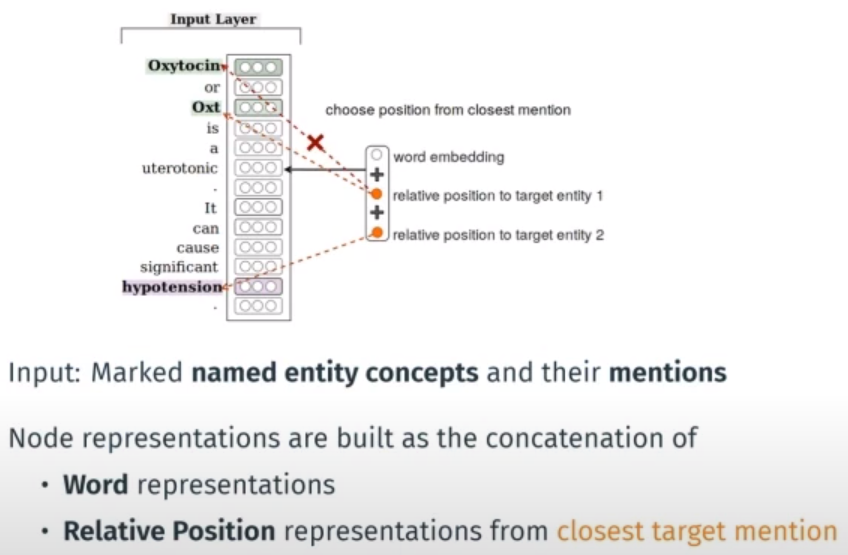Graph Construction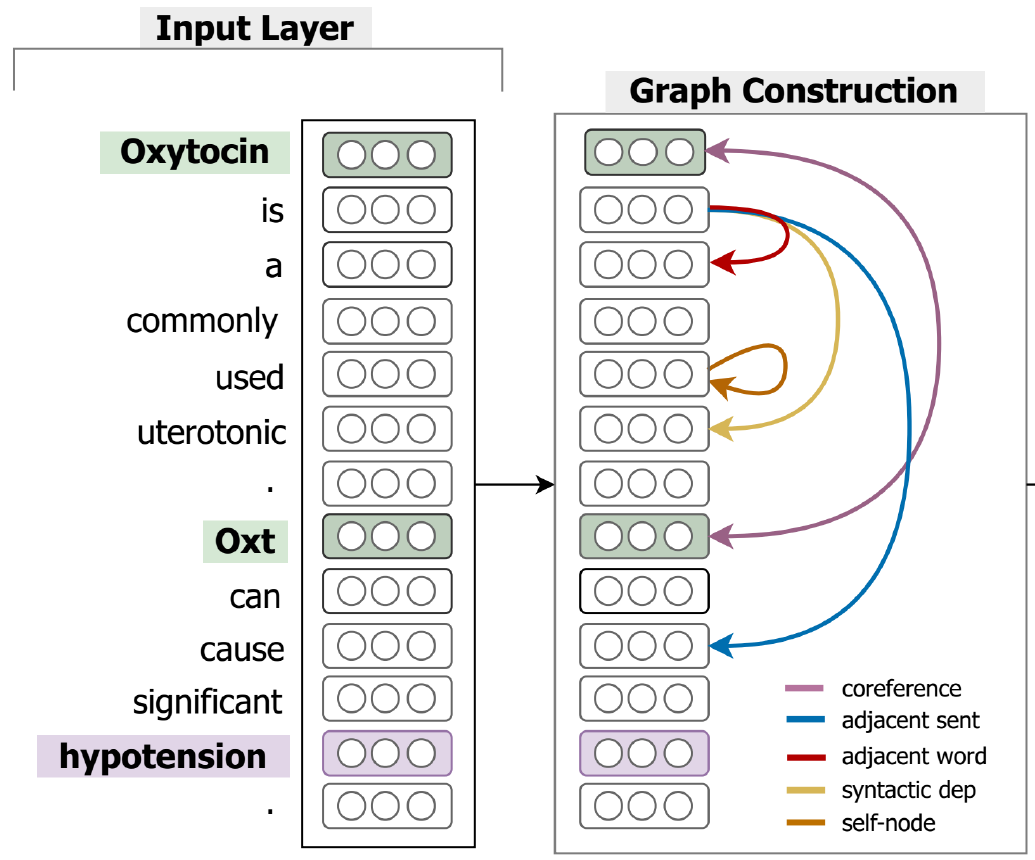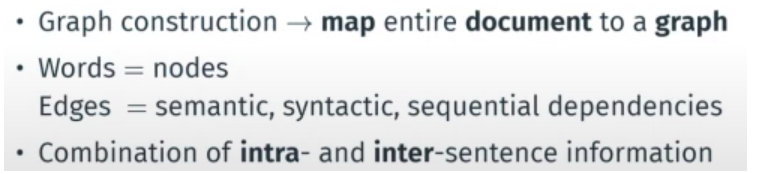local dependencies:

1. Syntactic dependency edge: (clues for intra-sentence relations) 句子内的句法依赖边。使用句子内的依存语法树，每种依存关系作为一种类型的边;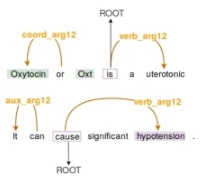combine the next word and the previous word to the current word in order to encode some kind of sequential information into the model.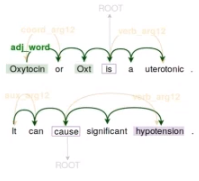1. Self-node edge: 自节点边，为了学习到自身的信息。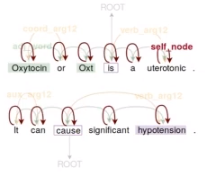non-local dependencies:

1. Coreference edge:共指边,代表两个指称描述同一个实体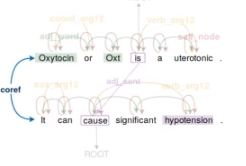1. Adjacent sentence edge:将相邻句子的依存语法树==根节点连接==构成一种类型的边（the root of sentence）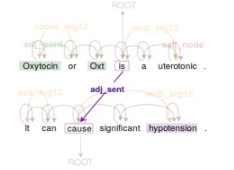GCNN Layer

GCNN使用的GCN和普通的GCN有一些不同，它只交互一阶相邻节点的信息，并且有K个块，==每个块针对一种边进行卷积操作==，最后将各个块的结果累加。

GCNN的整个处理过程其实可以看作是基于==5种关联类型构建了5个图==，分别进行图卷积操作，然后将5个图的结果累加。（怎么叠加呢）这样做的原因是不同的关联类型代表的含义是不同的，必须加以区分。（异质图？）GCNN最大的亮点就在这里，==通过分层巧妙的使用了多种关联关系。==

A labelled edge GCNN,keeping ==separate parameters for each edge type.==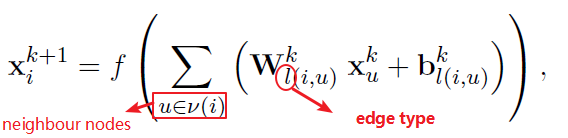$\mathbf{W}_{l(i, u)}^{k}$和$\mathbf{b}_{l(i, u)}^{k}$，其中$k$表示$k$-th block for ==edge type $l$,== between nodes $i$ and $u$ .

Stack $K$ GCNN blocks 目的：汇聚来自 distant neighbouring nodes的 information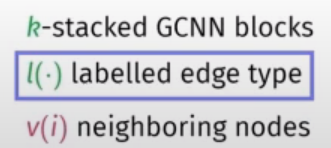In order to ==avoid over parameterization,==we tune the number of parameters and keep the top and keep most frequent edge types while merging all the remaining types as a single rare edge type.(有问题？？作者的意思就是五个不同的W吗？那最后x的信息怎么汇聚呢？五个不同的x呢呀)

A：应该是直接叠加

#### Tune number of parameters keeping top-N most frequent type & merging rare

MIL-based Relation Classification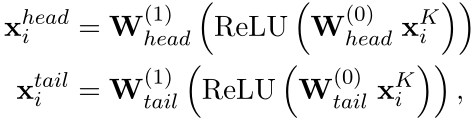$\mathbf{W}^{(1)}$和$\mathbf{W}^{(0)}$是分别是2-layer FFNNs的参数。$\mathrm{x}_{i}^{\text {head}}, \mathrm{x}_{i}^{\text {tail}} \in \mathbb{R}^{d}$

Aggregate mention pairs (x) —>>>>concept pair (e)，combine information form multiple mention level pairs into a single concept level pair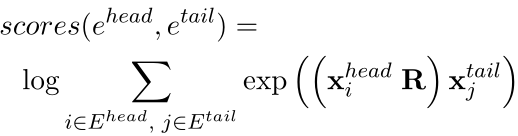$\mathbf{R} \in \mathbb{R}^{d \times r \times d}$ is a learned bi-affine tensor with $r$ ($r$ is the number of relation categories)

### Experiment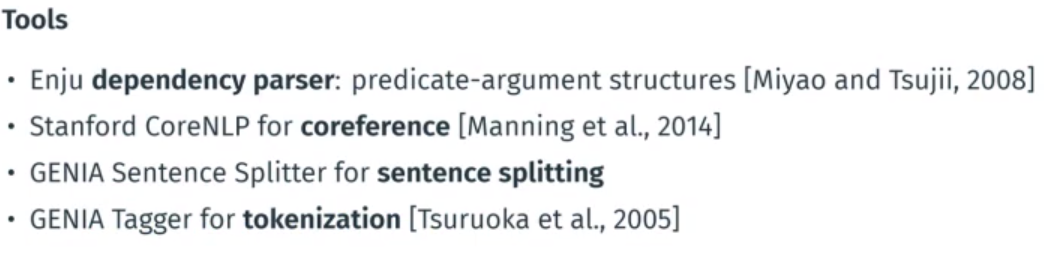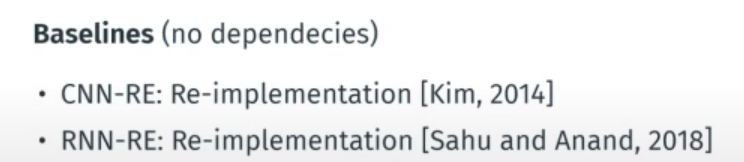CDR&CHR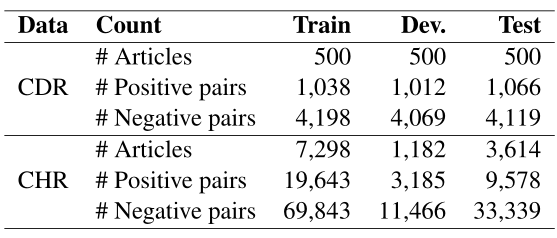​ Statistics of the CDR and CHR datasets.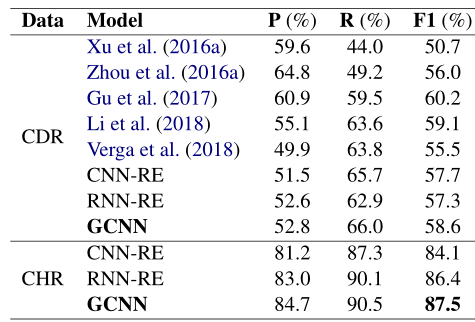​ Performance on the CDR and CHR test sets in comparison

What is the optimal number of edge types ?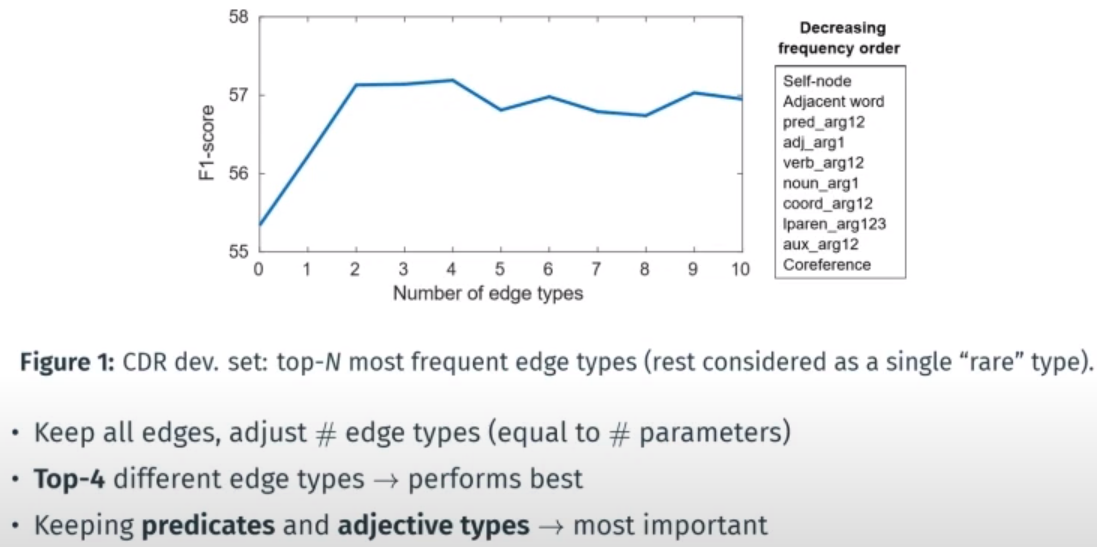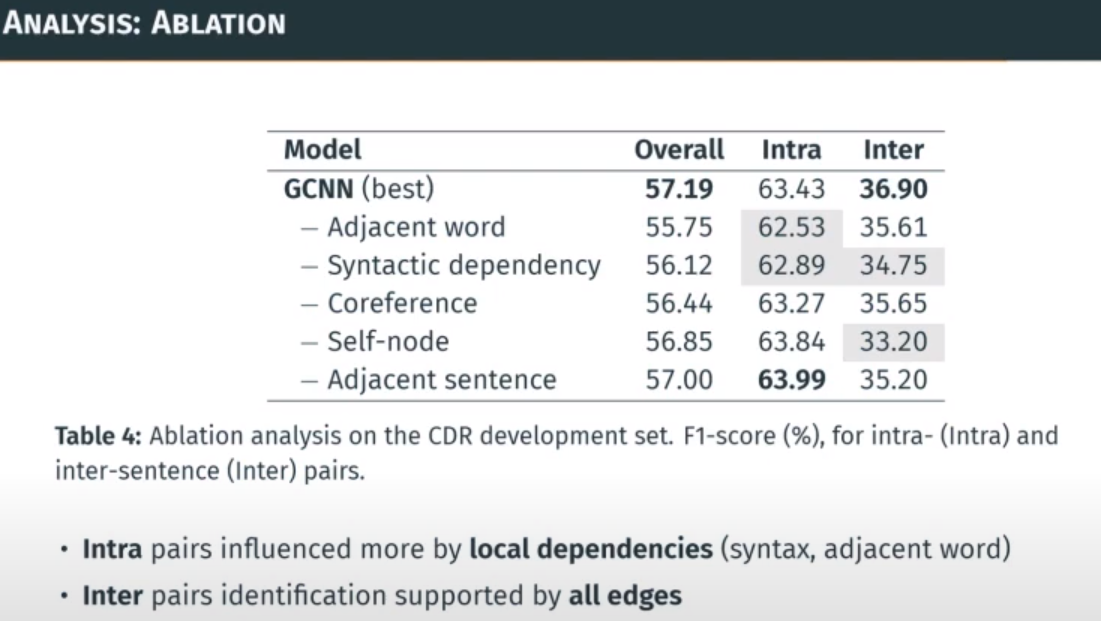GCNN 中规中矩，没有特别出色的地方：

logical reasoning几乎没有单独拿来讨论处理，纯靠GCNN（邻域聚合能推理个毛线？）；